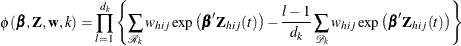Partial Likelihood Function for the Cox Model

Let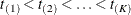denote the K distinct, ordered event times. Let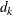denote the multiplicity of failures at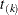; that is,is the size of the set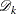of individuals that fail at. Let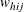be the weight associated with the jth observation unit in the ith cluster in stratum h. Using this notation, the pseudo-likelihood functions used in PROC SURVEYPHREG to estimate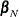are described in the following sections.

Continuous Time Scale

Let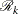denote the risk set just before the kth ordered event time.

The Breslow likelihood is expressed as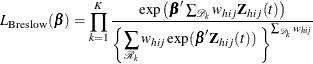The Efron likelihood is expressed as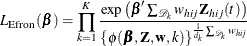where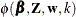is